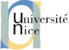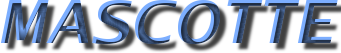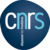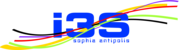## MASCOTTE no longer exists => visit the new COATI project-team

Publications of year 2013
BACK TO MASCOTTE PUBLICATION INDEX

## Publications of year 2013

 Articles in journal or book chapters
1. L. Addario-Berry, F. Havet, C. Linhares Sales, B. Reed, and S. Thomassé. Oriented trees in digraphs. Discrete Mathematics, 313(8):967-974, 2013. [WWW ] [PDF ]
Abstract:
"Let $f(k)$ be the smallest integer such that every $f(k)$-chromatic digraph contains every oriented tree of order $k$. Burr proved $f(k)\leq (k-1)^2$ in general, and he conjectured $f(k)=2k-2$. Burr also proved that every $(8k-7)$-chromatic digraph contains every antidirected tree. We improve both of Burr's bounds. We show that $f(k)\leq k^2/2-k/2+1$ and that every antidirected tree of order $k$ is contained in every $(5k-9)$-chromatic digraph. We make a conjecture that explains why antidirected trees are easier to handle. It states that if $|E(D)| > (k-2) |V(D)|$, then the digraph $D$ contains every antidirected tree of order $k$. This is a common strengthening of both Burr's conjecture for antidirected trees and the celebrated Erd\H{o}s-S\'os Conjecture. The analogue of our conjecture for general trees is false, no matter what function $f(k)$ is used in place of $k-2$. We prove our conjecture for antidirected trees of diameter 3 and present some other evidence for it. Along the way, we show that every acyclic $k$-chromatic digraph contains every oriented tree of order $k$ and suggest a number of approaches for making further progress on Burr's conjecture."

@article{AHL+13,
author = {Addario-Berry, L. and Havet, F. and Linhares Sales, C. and Reed, B. and Thomass\'e, S.},
title = {Oriented trees in digraphs},
JOURNAL = "Discrete Mathematics",
year = "2013",
VOLUME = "313",
number = "8",
PAGES = "967-974",
abstract = "Let $f(k)$ be the smallest integer such that every $f(k)$-chromatic digraph contains every oriented tree of order $k$. Burr proved $f(k)\leq (k-1)^2$ in general, and he conjectured $f(k)=2k-2$. Burr also proved that every $(8k-7)$-chromatic digraph contains every antidirected tree. We improve both of Burr's bounds. We show that $f(k)\leq k^2/2-k/2+1$ and that every antidirected tree of order $k$ is contained in every $(5k-9)$-chromatic digraph.

We make a conjecture that explains why antidirected trees are easier to handle. It states that if $|E(D)| > (k-2) |V(D)|$, then the digraph $D$ contains every antidirected tree of order $k$. This is a common strengthening of both Burr's conjecture for antidirected trees and the celebrated Erd\H{o}s-S\'os Conjecture. The analogue of our conjecture for general trees is false, no matter what function $f(k)$ is used in place of $k-2$. We prove our conjecture for antidirected trees of diameter 3 and present some other evidence for it.

Along the way, we show that every acyclic $k$-chromatic digraph contains every oriented tree of order $k$ and suggest a number of approaches for making further progress on Burr's conjecture.",
issn = "0012-365X",
doi = "10.1016/j.disc.2013.01.011",
url = "http://www.sciencedirect.com/science/article/pii/S0012365X13000289",
PDF = {http://hal.inria.fr/docs/00/55/11/33/PDF/RR-7502.pdf},
x-editorial-board={yes},
x-proceedings={yes},
x-international-audience={yes},
x-pays={BR,CA},
sorte = "rev-int",

}


2. J. Araujo, V. Campos, F. Giroire, N. Nisse, L. Sampaio, and R. Soares. On the hull number of some graph classes. Theoretical Computer Science, 475:1-12, 2013. [WWW ] [PDF ]
Abstract:
In this paper, we study the geodetic convexity of graphs focusing on the problem of the complexity to compute inclusion-minimum hull set of a graph in several graph classes. For any two vertices $u,v\in V$ of a connected graph $G=(V,E)$, the {\em closed interval} $I[u,v]$ of $u$ and $v$ is the the set of vertices that belong to some shortest $(u,v)$-path. For any $S \subseteq V$, let $I[S]= \bigcup\_{u,v\in S} I[u,v]$. A subset $S\subseteq V$ is {\em geodesically convex} if $I[S] = S$. In other words, a subset $S$ is convex if, for any $u,v \in S$ and for any shortest $(u,v)$-path $P$, $V(P) \subseteq S$. Given a subset $S\subseteq V$, the {\em convex hull} $I\_h[S]$ of $S$ is the smallest convex set that contains $S$. We say that $S$ is a {\em hull set} of $G$ if $I\_h[S] = V$. The size of a minimum hull set of $G$ is the {\em hull number} of $G$, denoted by $hn(G)$. The {\sc Hull Number} problem is to decide whether $hn(G)\leq k$, for a given graph $G$ and an integer $k$. Dourado {\it et al.} showed that this problem is NP-complete in general graphs. In this paper, we answer an open question of Dourado et al.\~\cite{Douradoetal09} by showing that the {\sc Hull Number} problem is NP-hard even when restricted to the class of bipartite graphs. Then, we design polynomial time algorithms to solve the {\sc Hull Number} problem in several graph classes. First, we deal with the class of complements of bipartite graphs. Then, we generalize some results in\~\cite{ACGSS11} to the class of $(q,q-4)$-graphs and to the class of cacti. Finally, we prove tight upper bounds on the hull numbers. In particular, we show that the hull number of an $n$-node graph $G$ without simplicial vertices is at most $1+\lceil \frac{3(n-1)}{5}\rceil$ in general, at most $1+\lceil \frac{n-1}{2}\rceil$ if $G$ is regular or has no triangle, and at most $1+\lceil \frac{n-1}{3}\rceil$ if $G$ has girth at least $6$.

@article{ACG+13,
author = {Araujo, J. and Campos, V. and Giroire, F. and Nisse, N. and Sampaio, L. and Soares, R.},
title = {{On the hull number of some graph classes}},
journal = {Theoretical Computer Science},
year = {2013},
volume = {475},
pages = {1-12},
abstract = {{In this paper, we study the geodetic convexity of graphs focusing on the problem of the complexity to compute inclusion-minimum hull set of a graph in several graph classes. For any two vertices $u,v\in V$ of a connected graph $G=(V,E)$, the {\em closed interval} $I[u,v]$ of $u$ and $v$ is the the set of vertices that belong to some shortest $(u,v)$-path. For any $S \subseteq V$, let $I[S]= \bigcup\_{u,v\in S} I[u,v]$. A subset $S\subseteq V$ is {\em geodesically convex} if $I[S] = S$. In other words, a subset $S$ is convex if, for any $u,v \in S$ and for any shortest $(u,v)$-path $P$, $V(P) \subseteq S$. Given a subset $S\subseteq V$, the {\em convex hull} $I\_h[S]$ of $S$ is the smallest convex set that contains $S$. We say that $S$ is a {\em hull set} of $G$ if $I\_h[S] = V$. The size of a minimum hull set of $G$ is the {\em hull number} of $G$, denoted by $hn(G)$. The {\sc Hull Number} problem is to decide whether $hn(G)\leq k$, for a given graph $G$ and an integer $k$. Dourado {\it et al.} showed that this problem is NP-complete in general graphs. In this paper, we answer an open question of Dourado et al.\~\cite{Douradoetal09} by showing that the {\sc Hull Number} problem is NP-hard even when restricted to the class of bipartite graphs. Then, we design polynomial time algorithms to solve the {\sc Hull Number} problem in several graph classes. First, we deal with the class of complements of bipartite graphs. Then, we generalize some results in\~\cite{ACGSS11} to the class of $(q,q-4)$-graphs and to the class of cacti. Finally, we prove tight upper bounds on the hull numbers. In particular, we show that the hull number of an $n$-node graph $G$ without simplicial vertices is at most $1+\lceil \frac{3(n-1)}{5}\rceil$ in general, at most $1+\lceil \frac{n-1}{2}\rceil$ if $G$ is regular or has no triangle, and at most $1+\lceil \frac{n-1}{3}\rceil$ if $G$ has girth at least $6$.}},
url = {http://hal.inria.fr/inria-00576581/en/},
pdf = {http://hal.inria.fr/inria-00576581/PDF/hn-RR\_v2.pdf},
x-editorial-board={yes},
x-proceedings={no},
x-international-audience={yes},
x-pays = {BR},
sorte ="rev-int",

}


3. Jean-Claude Bermond, Michel Cosnard, and Stéphane Pérennes. Directed acyclic graphs with the unique dipath property. Theoretical Computer Science, 504:5-11, September 2013. [WWW ] [PDF ]
Keywords: DAG (Directed acyclic graphs), load, wavelengths, dipaths, good labelings, conflict graphs, intersection graphs, chromatic number.
Abstract:
Let P be a family of dipaths of a DAG (Directed Acyclic Graph) G. The load of an arc is the number of dipaths containing this arc. Let $\pi$(G, P) be the maximum of the load of all the arcs and let w(G, P) be the minimum number of wavelengths (colors) needed to color the family of dipaths P in such a way that two dipaths with the same wavelength are arc-disjoint. There exist DAGs such that the ratio between w(G, P) and $\pi$(G, P) cannot be bounded. An internal cycle is an oriented cycle such that all the vertices have at least one predecessor and one successor in G (said otherwise every cycle contain neither a source nor a sink of G). We prove that, for any family of dipaths P, w(G, P) = $\pi$(G, P) if and only if G is without internal cycle. We also consider a new class of DAGs, which is of interest in itself, those for which there is at most one dipath from a vertex to another. We call these digraphs UPP-DAGs. For these UPP-DAGs we show that the load is equal to the maximum size of a clique of the conflict graph. We prove that the ratio between w(G, P) and $\pi$(G, P) cannot be bounded (a result conjectured in an other article). For that we introduce "good labelings" of the conflict graph associated to G and P, namely labelings of the edges such that for any ordered pair of vertices (x, y) there do not exist two paths from x to y with increasing labels.

@article{bermond:hal-00869501,
hal_id = {hal-00869501},
url = {http://hal.archives-ouvertes.fr/hal-00869501},
title = {{Directed acyclic graphs with the unique dipath property}},
author = {Bermond, Jean-Claude and Cosnard, Michel and P{\'e}rennes, St{\'e}phane},
abstract = {{Let P be a family of dipaths of a DAG (Directed Acyclic Graph) G. The load of an arc is the number of dipaths containing this arc. Let $\pi$(G, P) be the maximum of the load of all the arcs and let w(G, P) be the minimum number of wavelengths (colors) needed to color the family of dipaths P in such a way that two dipaths with the same wavelength are arc-disjoint. There exist DAGs such that the ratio between w(G, P) and $\pi$(G, P) cannot be bounded. An internal cycle is an oriented cycle such that all the vertices have at least one predecessor and one successor in G (said otherwise every cycle contain neither a source nor a sink of G). We prove that, for any family of dipaths P, w(G, P) = $\pi$(G, P) if and only if G is without internal cycle. We also consider a new class of DAGs, which is of interest in itself, those for which there is at most one dipath from a vertex to another. We call these digraphs UPP-DAGs. For these UPP-DAGs we show that the load is equal to the maximum size of a clique of the conflict graph. We prove that the ratio between w(G, P) and $\pi$(G, P) cannot be bounded (a result conjectured in an other article). For that we introduce "good labelings" of the conflict graph associated to G and P, namely labelings of the edges such that for any ordered pair of vertices (x, y) there do not exist two paths from x to y with increasing labels.}},
keywords = {DAG (Directed acyclic graphs); load; wavelengths; dipaths; good labelings; conflict graphs; intersection graphs; chromatic number},
language = {Anglais},
affiliation = {COATI - Inria Sophia Antipolis / Laboratoire I3S , MASCOTTE - INRIA Sophia Antipolis / Laboratoire I3S},
pages = {5-11},
journal = {Theoretical Computer Science},
publisher = {Elsevier},
volume = {504},
audience = {internationale },
doi = {10.1016/j.tcs.2012.06.015 },
year = {2013},
month = Sep,
pdf = {http://hal.archives-ouvertes.fr/hal-00869501/PDF/tcs270412.pdf},

}


4. S. Guillemot, F. Havet, C. Paul, and A. Perez. On the (non-)existence of polynomial kernels for $P_l$-free edge modification problems. Algorithmica, 65(4):900-926, 2013. [PDF ]
Abstract:
Given a graph $G=(V,E)$ and a positive integer $k$, an edge modification problem for a graph property $\Pi$ consists in deciding whether there exists a set $F$ of pairs of $V$ of size at most $k$ such that the graph $H=(V,E\vartriangle F)$ satisfies the property $\Pi$. In the $\Pi$ \emph{edge-completion problem}, the set $F$ is constrained to be disjoint from $E$; in the $\Pi$ \emph{edge-deletion problem}, $F$ is a subset of $E$; no constraint is imposed on $F$ in the $\Pi$ \emph{edge-editing problem}. A number of optimization problems can be expressed in terms of graph modification problems which have been extensively studied in the context of parameterized complexity. When parameterized by the size $k$ of the set $F$, it has been proved that if $\Pi$ is an hereditary property characterized by a finite set of forbidden induced subgraphs, then the three $\Pi$ edge-modification problems are FPT. It was then natural to ask whether these problems also admit a polynomial kernel. Using recent lower bound techniques, Kratsch and Wahlstr\"om answered this question negatively. However, the problem remains open on many natural graph classes characterized by forbidden induced subgraphs. Kratsch and Wahlstr\"om asked whether the result holds when the forbidden subgraphs are paths or cycles and pointed out that the problem is already open in the case of $P_4$-free graphs (i.e. cographs). This paper provides positive and negative results in that line of research. We prove that \textsc{Parameterized cograph edge-modification} problems have cubic vertex kernels whereas polynomial kernels are unlikely to exist for the \textsc{$P_l$-free edge-deletion} and the \textsc{$C_l$-free edge-deletion} problems for $l\geq 7$ and $l\geq 4$ respectively. Indeed, if they exist, then $NP \subseteq coNP / poly$.

@article{GHP+13,
AUTHOR="Guillemot, S. and Havet, F. and Paul, C. and Perez, A.",
TITLE="On the (non-)existence of polynomial kernels for $P_l$-free edge modification problems",
JOURNAL = "Algorithmica",
year = "2013",
VOLUME = "65",
number ="4",
PAGES = "900-926",
abstract = {Given a graph $G=(V,E)$ and a positive integer $k$, an edge modification problem for a graph property $\Pi$ consists in deciding whether there exists a set $F$ of pairs of $V$ of size at most $k$ such that the graph $H=(V,E\vartriangle F)$ satisfies the property $\Pi$. In the $\Pi$ \emph{edge-completion problem}, the set $F$ is constrained to be disjoint from $E$; in the $\Pi$ \emph{edge-deletion problem}, $F$ is a subset of $E$; no constraint is imposed on $F$ in the $\Pi$ \emph{edge-editing problem}. A number of optimization problems can be expressed in terms of graph modification problems which have been extensively studied in the context of parameterized complexity. When parameterized by the size $k$ of the set $F$, it has been proved that if $\Pi$ is an hereditary property characterized by a finite set of forbidden induced subgraphs, then the three $\Pi$ edge-modification problems are FPT. It was then natural to ask whether these problems also admit a polynomial kernel. Using recent lower bound techniques, Kratsch and Wahlstr\"om answered this question negatively. However, the problem remains open on many natural graph classes characterized by forbidden induced subgraphs. Kratsch and Wahlstr\"om asked whether the result holds when the forbidden subgraphs are paths or cycles and pointed out that the problem is already open in the case of $P_4$-free graphs (i.e. cographs). This paper provides positive and negative results in that line of research. We prove that \textsc{Parameterized cograph edge-modification} problems have cubic vertex kernels whereas polynomial kernels are unlikely to exist for the \textsc{$P_l$-free edge-deletion} and the \textsc{$C_l$-free edge-deletion} problems for $l\geq 7$ and $l\geq 4$ respectively. Indeed, if they exist, then $NP \subseteq coNP / poly$.},
pdf = {ftp://ftp-sop.inria.fr/coati/Publications/GHP+13.pdf},
OPTx-editorial-board={yes},
OPTx-proceedings={no},
OPTx-international-audience={yes},
x-pays = {},
sorte = "rev-int",

}


BACK TO MASCOTTE PUBLICATION INDEX